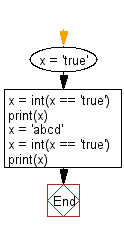﻿ Python: Convert true to 1 and false to 0 - w3resource# Python: Convert true to 1 and false to 0

## Python Basic: Exercise-138 with Solution

Write a Python program to convert true to 1 and false to 0.

Sample Solution:-

Python Code:

``````x = 'true'
x = int(x == 'true')
print(x)
x = 'abcd'
x = int(x == 'true')
print(x)
```
```

Sample Output:

```1
0
```

Flowchart:## Visualize Python code execution:

The following tool visualize what the computer is doing step-by-step as it executes the said program:

Python Code Editor:

Have another way to solve this solution? Contribute your code (and comments) through Disqus.

What is the difficulty level of this exercise?

Test your Python skills with w3resource's quiz

﻿

## Python: Tips of the Day

Try-catch-else construct:

```try:
foo()
except Exception:
print("Exception occured")
else:
print("Exception didnt occur")
finally:
print("Always gets here")
```